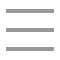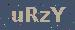个人随笔

leetcode(六)、回文数
2021-06-29 18:29:39

-231 <= x <= 231 - 1

#### 解题要点

``public class Test8 {``    /**``     * 这里直接当作是字符串处理即可,难度系数完全比不上回文子串:简单是简单，为啥击败的人这么少，感觉已经是极致了呀``     * 执行用时：20 ms, 在所有 Java 提交中击败了6.98%的用户内存消耗：37.7 MB, 在所有 Java 提交中击败了75.97%的用户``     */``    public boolean isPalindrome(int x) {``        String str = x+"";``        //二分即可``        int length = str.length();``        if(length==1) {``            return true;``        }``        int left=0;``        int right = length-1;``        while(left<right){``            //回文的特性就是第一位等于最后一位，以此类推即可``            if(str.charAt(left++)!=str.charAt(right--)) {``                //如果不等于``                return false;``            }``        }``        return true;``    }``    public static void main(String[] args) {``        System.out.println(new Test8().isPalindrome(121));``        System.out.println(new Test8().isPalindrome(-121));``        System.out.println(new Test8().isPalindrome(10));``        System.out.println(new Test8().isPalindrome(-101));``        System.out.println(new Test8().isPalindrome(11));``    }``}``

13Copyright : 个人随笔   备案号 : 粤ICP备18099399号